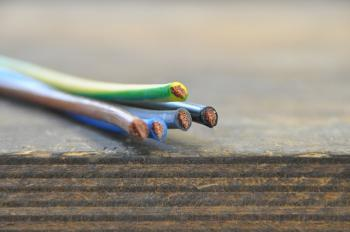# What is electricity?The electric current is the flow of electric charge passing through a material per unit of time. Moving electrical charges produce a magnetic field.

It is called electrical charge to the physical property that possess certain subatomic particles (like the protons and the electrons). This property manifests manifested through the forces of repulsion and attraction that exist between them by means of electromagnetic fields. Protons have a positive charge and negatively charged electrons. This assignment of charges was performed arbitrarily.

Anyway, when we talk about electric current, these electric charges are usually electrons.

For the electric charge to move it must be subjected to a potential difference.

## How is the electric current measured?

From the electric current, two quantities are defined:

• Current
• Current density

The current intensity (I) in a given section of a conductor (s) is defined as the electric charge (Q) that crosses the section in a unit of time (t):

Current density (j) is the current flowing through a section per unit area of section (S).

In the international system of units the electric current is expressed in coulombs per second (C / s), that is, in Amperes (A).

The galvanometer is the instrument to measure the intensity of an electric current. The galvanometer that has been calibrated in amps is called an ammeter.

## History of electric current

Initially the electric current was defined as a flow of positive charges. The conventional sense of flow of the current was fixed as a flow of charges from the positive to the negative pole.

Later, thanks to the Hall effect, it was observed that in the metals the charge carriers were electrons, with negative charge. Electrons move in the opposite direction. This finding contradicted the above, since the electrons would move from the negative pole to the positive pole.

The first experiments with electricity were made in the eighteenth century. At that time, only electric charge generated by rubbing (static electricity) or by induction was available. The first movement of constant charge was not achieved until 1800 thanks to the first electric battery invented by the Italian physicist Alessandro Volta.

valoración: 3 - votos 1

Last review: March 13, 2017

Back